# P. Aschieri - Noncommutative Differential Geometry: Quantization of Connections and Gravity

Sep. 10, 2011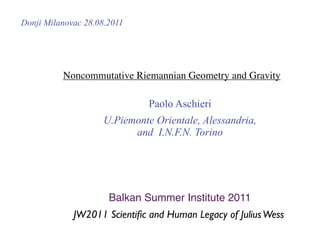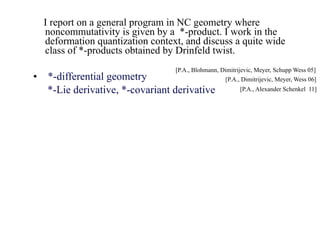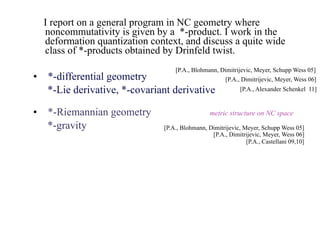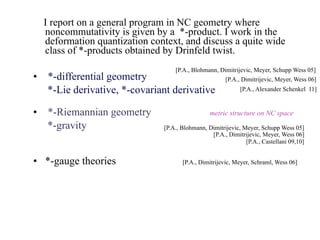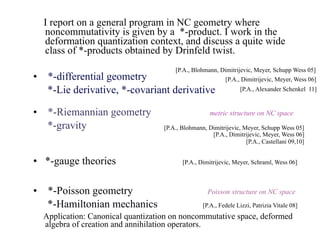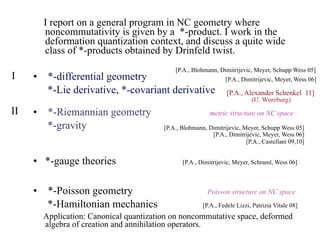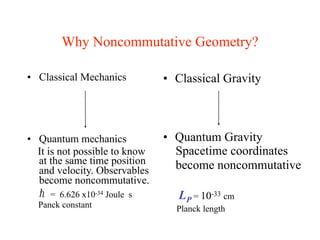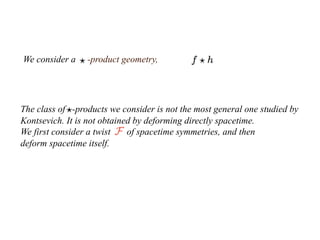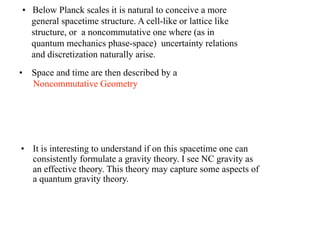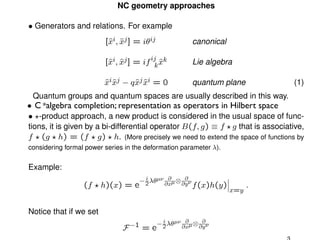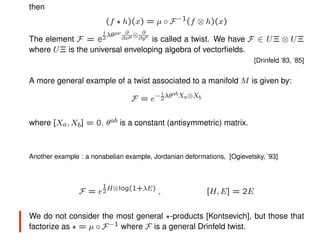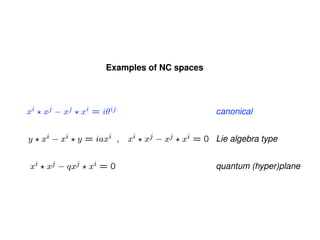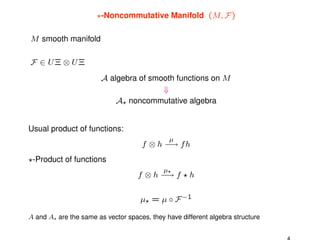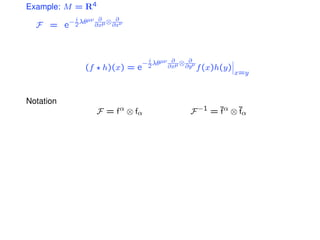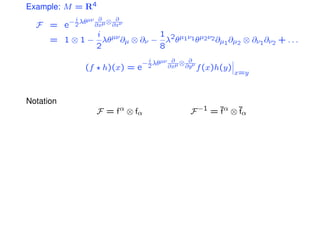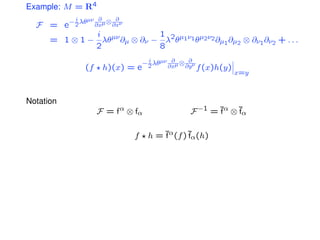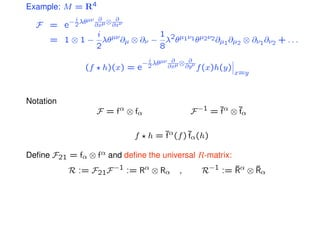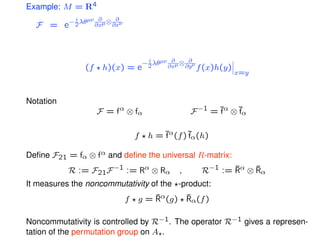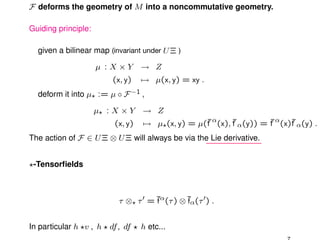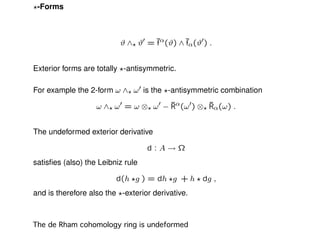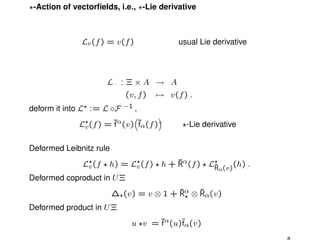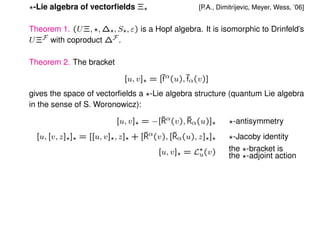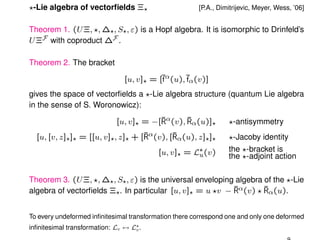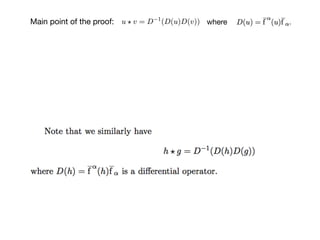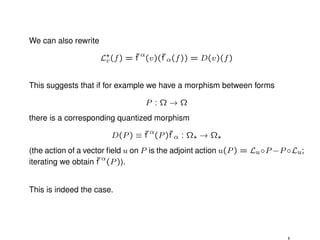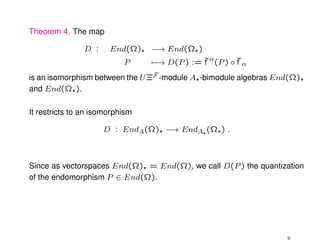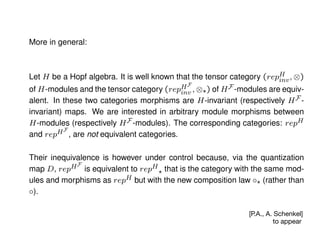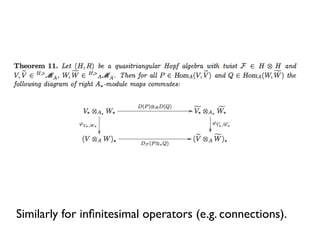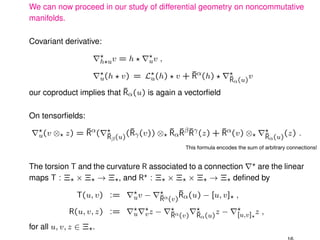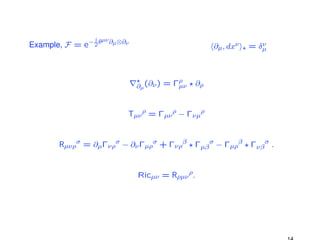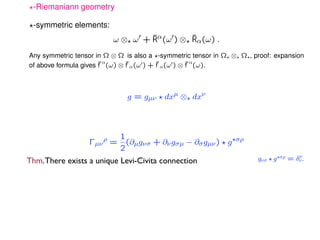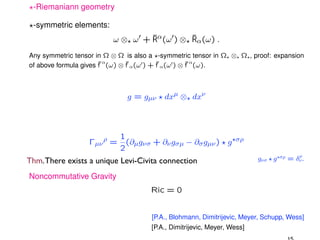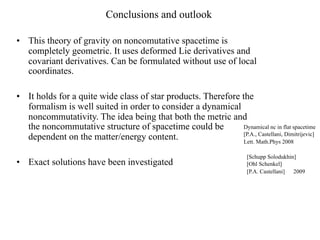1 of 33

### P. Aschieri - Noncommutative Differential Geometry: Quantization of Connections and Gravity

• 1. Donji Milanovac 28.08.2011 Noncommutative Riemannian Geometry and Gravity Paolo Aschieri U.Piemonte Orientale, Alessandria, and I.N.F.N. Torino Balkan Summer Institute 2011 JW2011 Scientiﬁc and Human Legacy of Julius Wess
• 2. I report on a general program in NC geometry where noncommutativity is given by a *-product. I work in the deformation quantization context, and discuss a quite wide class of *-products obtained by Drinfeld twist. [P.A., Blohmann, Dimitrijevic, Meyer, Schupp Wess 05] • *-differential geometry [P.A., Dimitrijevic, Meyer, Wess 06] *-Lie derivative, *-covariant derivative [P.A., Alexander Schenkel 11]
• 3. I report on a general program in NC geometry where noncommutativity is given by a *-product. I work in the deformation quantization context, and discuss a quite wide class of *-products obtained by Drinfeld twist. [P.A., Blohmann, Dimitrijevic, Meyer, Schupp Wess 05] • *-differential geometry [P.A., Dimitrijevic, Meyer, Wess 06] *-Lie derivative, *-covariant derivative [P.A., Alexander Schenkel 11] • *-Riemannian geometry metric structure on NC space *-gravity [P.A., Blohmann, Dimitrijevic, Meyer, Schupp Wess 05] [P.A., Dimitrijevic, Meyer, Wess 06] [P.A., Castellani 09,10]
• 4. I report on a general program in NC geometry where noncommutativity is given by a *-product. I work in the deformation quantization context, and discuss a quite wide class of *-products obtained by Drinfeld twist. [P.A., Blohmann, Dimitrijevic, Meyer, Schupp Wess 05] • *-differential geometry [P.A., Dimitrijevic, Meyer, Wess 06] *-Lie derivative, *-covariant derivative [P.A., Alexander Schenkel 11] • *-Riemannian geometry metric structure on NC space *-gravity [P.A., Blohmann, Dimitrijevic, Meyer, Schupp Wess 05] [P.A., Dimitrijevic, Meyer, Wess 06] [P.A., Castellani 09,10] • *-gauge theories [P.A., Dimitrijevic, Meyer, Schraml, Wess 06]
• 5. I report on a general program in NC geometry where noncommutativity is given by a *-product. I work in the deformation quantization context, and discuss a quite wide class of *-products obtained by Drinfeld twist. [P.A., Blohmann, Dimitrijevic, Meyer, Schupp Wess 05] • *-differential geometry [P.A., Dimitrijevic, Meyer, Wess 06] *-Lie derivative, *-covariant derivative [P.A., Alexander Schenkel 11] • *-Riemannian geometry metric structure on NC space *-gravity [P.A., Blohmann, Dimitrijevic, Meyer, Schupp Wess 05] [P.A., Dimitrijevic, Meyer, Wess 06] [P.A., Castellani 09,10] • *-gauge theories [P.A., Dimitrijevic, Meyer, Schraml, Wess 06] • *-Poisson geometry Poisson structure on NC space *-Hamiltonian mechanics [P.A., Fedele Lizzi, Patrizia Vitale 08] Application: Canonical quantization on noncommutative space, deformed algebra of creation and annihilation operators.
• 6. I report on a general program in NC geometry where noncommutativity is given by a *-product. I work in the deformation quantization context, and discuss a quite wide class of *-products obtained by Drinfeld twist. [P.A., Blohmann, Dimitrijevic, Meyer, Schupp Wess 05] I • *-differential geometry [P.A., Dimitrijevic, Meyer, Wess 06] *-Lie derivative, *-covariant derivative [P.A., Alexander Schenkel 11] (U. Wurzburg) II • *-Riemannian geometry metric structure on NC space *-gravity [P.A., Blohmann, Dimitrijevic, Meyer, Schupp Wess 05] [P.A., Dimitrijevic, Meyer, Wess 06] [P.A., Castellani 09,10] • *-gauge theories [P.A., Dimitrijevic, Meyer, Schraml, Wess 06] • *-Poisson geometry Poisson structure on NC space *-Hamiltonian mechanics [P.A., Fedele Lizzi, Patrizia Vitale 08] Application: Canonical quantization on noncommutative space, deformed algebra of creation and annihilation operators.
• 7. Why Noncommutative Geometry? • Classical Mechanics • Classical Gravity • Quantum mechanics • Quantum Gravity It is not possible to know Spacetime coordinates at the same time position become noncommutative and velocity. Observables become noncommutative. = 6.626 x10-34 Joule s LP = 10-33 cm Panck constant Planck length
• 8. We consider a -product geometry, The class of -products we consider is not the most general one studied by Kontsevich. It is not obtained by deforming directly spacetime. We first consider a twist of spacetime symmetries, and then deform spacetime itself.
• 9. • Below Planck scales it is natural to conceive a more general spacetime structure. A cell-like or lattice like structure, or a noncommutative one where (as in quantum mechanics phase-space) uncertainty relations and discretization naturally arise. • Space and time are then described by a Noncommutative Geometry • It is interesting to understand if on this spacetime one can consistently formulate a gravity theory. I see NC gravity as an effective theory. This theory may capture some aspects of a quantum gravity theory.
• 10. NC geometry approaches • Generators and relations. For example [ˆi, xj ] = iθij x ˆ canonical ij [ˆi, xj ] = if k xk x ˆ ˆ Lie algebra xixj − qˆj xi = 0 ˆˆ x ˆ quantum plane (1) Quantum groups and quantum spaces are usually described in this way. C *algebra completion; representation as operators in Hilbert space • -product approach, a new product is considered in the usual space of func- tions, it is given by a bi-differential operator B(f, g) ≡ f g that is associative, f (g h) = (f g) h. (More precisely we need to extend the space of functions by considering formal power series in the deformation parameter λ). Example: i ∂ ∂ − 2 λθµν ∂xµ ⊗ ∂yν (f h)(x) = e f (x)h(y) . x=y Notice that if we set i µν ∂ ∂ F −1 = e− 2 λθ ∂xµ ⊗ ∂yν
• 11. then (f h)(x) = µ ◦ F −1(f ⊗ h)(x) i µν ∂ ∂ The element F = e 2 λθ ∂xµ ⊗ ∂y ν is called a twist. We have F ∈ U Ξ ⊗ U Ξ where U Ξ is the universal enveloping algebra of vectorﬁelds. [Drinfeld ’83, ’85] A more general example of a twist associated to a manifold M is given by: i ab F = e− 2 λθ Xa⊗Xb where [Xa, Xb] = 0. θab is a constant (antisymmetric) matrix. Another example : a nonabelian example, Jordanian deformations, [Ogievetsky, ’93] 1 H⊗log(1+λE) F= e2 , [H, E] = 2E | We do not consider the most general -products [Kontsevich], but those that factorize as = µ ◦ F −1 where F is a general Drinfeld twist.
• 12. Examples of NC spaces xi xj − xj xi = iθij canonical y xi − xi y = iaxi , xi xj − xj xi = 0 Lie algebra type xi xj − qxj xi = 0 quantum (hyper)plane
• 13. -Noncommutative Manifold (M, F ) M smooth manifold F ∈ UΞ ⊗ UΞ A algebra of smooth functions on M ⇓ A noncommutative algebra Usual product of functions: µ f ⊗ h −→ f h -Product of functions µ f ⊗ h −→ f h µ = µ ◦ F −1 A and A are the same as vector spaces, they have different algebra structure
• 14. Example: M = R4 i ∂ ∂ − 2 λθµν ∂xµ ⊗ ∂xν F = e i 1 1 ⊗ 1 − λθµν ∂µ ⊗ ∂ν − λ2θµ1ν1 θµ2ν2 ∂µ1 ∂µ2 ⊗ ∂ν1 ∂ν2 + . . . 2 8 i ∂ ∂ − 2 λθµν ∂xµ ⊗ ∂yν (f h)(x) = e f (x)h(y) x=y Notation F = f α ⊗ fα F −1 = ¯α ⊗ ¯α f f f h = ¯α(f ) ¯α(h) f f Deﬁne F21 = fα ⊗ f α and deﬁne the universal R-matrix: R := F21F −1 := Rα ⊗ Rα , R−1 := Rα ⊗ Rα ¯ ¯ It measures the noncommutativity of the -product: ¯ ¯ f g = Rα(g) Rα(f ) Noncommutativity is controlled by R−1. The operator R−1 gives a represen- tation of the permutation group on A.
• 15. Example: M = R4 i ∂ ∂ − 2 λθµν ∂xµ ⊗ ∂xν F = e i 1 = 1 ⊗ 1 − λθµν ∂µ ⊗ ∂ν − λ2θµ1ν1 θµ2ν2 ∂µ1 ∂µ2 ⊗ ∂ν1 ∂ν2 + . . . 2 8 i ∂ ∂ − 2 λθµν ∂xµ ⊗ ∂yν (f h)(x) = e f (x)h(y) x=y Notation F = f α ⊗ fα F −1 = ¯α ⊗ ¯α f f f h = ¯α(f ) ¯α(h) f f Deﬁne F21 = fα ⊗ f α and deﬁne the universal R-matrix: R := F21F −1 := Rα ⊗ Rα , R−1 := Rα ⊗ Rα ¯ ¯ It measures the noncommutativity of the -product: ¯ ¯ f g = Rα(g) Rα(f ) Noncommutativity is controlled by R−1. The operator R−1 gives a represen- tation of the permutation group on A.
• 16. Example: M = R4 i ∂ ∂ − 2 λθµν ∂xµ ⊗ ∂xν F = e i 1 = 1 ⊗ 1 − λθµν ∂µ ⊗ ∂ν − λ2θµ1ν1 θµ2ν2 ∂µ1 ∂µ2 ⊗ ∂ν1 ∂ν2 + . . . 2 8 i ∂ ∂ − 2 λθµν ∂xµ ⊗ ∂yν (f h)(x) = e f (x)h(y) x=y Notation F = f α ⊗ fα F −1 = ¯α ⊗ ¯α f f f h = ¯α(f ) ¯α(h) f f Deﬁne F21 = fα ⊗ f α and deﬁne the universal R-matrix: R := F21F −1 := Rα ⊗ Rα , R−1 := Rα ⊗ Rα ¯ ¯ It measures the noncommutativity of the -product: ¯ ¯ f g = Rα(g) Rα(f ) Noncommutativity is controlled by R−1. The operator R−1 gives a represen- tation of the permutation group on A.
• 17. Example: M = R4 i ∂ ∂ − 2 λθµν ∂xµ ⊗ ∂xν F = e i 1 = 1 ⊗ 1 − λθµν ∂µ ⊗ ∂ν − λ2θµ1ν1 θµ2ν2 ∂µ1 ∂µ2 ⊗ ∂ν1 ∂ν2 + . . . 2 8 i ∂ ∂ − 2 λθµν ∂xµ ⊗ ∂yν (f h)(x) = e f (x)h(y) x=y Notation F = f α ⊗ fα F −1 = ¯α ⊗ ¯α f f f h = ¯α(f ) ¯α(h) f f Deﬁne F21 = fα ⊗ f α and deﬁne the universal R-matrix: R := F21F −1 := Rα ⊗ Rα , R−1 := Rα ⊗ Rα ¯ ¯ It measures the noncommutativity of the -product: ¯ ¯ f g = Rα(g) Rα(f ) Noncommutativity is controlled by R−1. The operator R−1 gives a represen- tation of the permutation group on A.
• 18. Example: M = R4 i ∂ ∂ − 2 λθµν ∂xµ ⊗ ∂xν F = e i 1 1 ⊗ 1 − λθµν ∂µ ⊗ ∂ν − λ2θµ1ν1 θµ2ν2 ∂µ1 ∂µ2 ⊗ ∂ν1 ∂ν2 + . . . 2 8 i ∂ ∂ − 2 λθµν ∂xµ ⊗ ∂yν (f h)(x) = e f (x)h(y) x=y Notation F = f α ⊗ fα F −1 = ¯α ⊗ ¯α f f f h = ¯α(f ) ¯α(h) f f Deﬁne F21 = fα ⊗ f α and deﬁne the universal R-matrix: R := F21F −1 := Rα ⊗ Rα , R−1 := Rα ⊗ Rα ¯ ¯ It measures the noncommutativity of the -product: ¯ ¯ f g = Rα(g) Rα(f ) Noncommutativity is controlled by R−1. The operator R−1 gives a represen- tation of the permutation group on A.
• 19. F deforms the geometry of M into a noncommutative geometry. Guiding principle: given a bilinear map (invariant under ) µ :X ×Y → Z (x, y) → µ(x, y) = xy . deform it into µ := µ ◦ F −1 , µ : X × Y → Z (x, y) → µ(x, y) = µ(f α(x), f α(y)) = f α(x)f α(y) . ¯ ¯ ¯ ¯ ________ The action of F ∈ U Ξ ⊗ U Ξ will always be via the Lie derivative. -Tensorﬁelds τ ⊗ τ = ¯α(τ ) ⊗ ¯α(τ ) . f f In particular h v , h df , df h etc...
• 20. -Forms ϑ ∧ ϑ = ¯α(ϑ) ∧ ¯α(ϑ) . f f Exterior forms are totally -antisymmetric. For example the 2-form ω ∧ ω is the -antisymmetric combination ω ∧ ω = ω ⊗ ω − Rα(ω ) ⊗ Rα(ω) . ¯ ¯ The undeformed exterior derivative d:A→Ω satisﬁes (also) the Leibniz rule d(h g ) = dh g + h dg , and is therefore also the -exterior derivative. The de Rham cohomology ring is undeformed. The de Rham cohomology ring is undeformed 8
• 21. -Action of vectorﬁelds, i.e., -Lie derivative Lv (f ) = v(f ) usual Lie derivative v Lv : Ξ × A → A (v, f ) → v(f ) . deform it into L := L ◦F −1 , (f ) = ¯α (v) ¯ (f ) _ Lv f fα -Lie derivative Deformed Leibnitz rule v v ¯ L (f h) = L (f ) h + Rα(f ) L (v)(h) . ¯ Rα Deformed coproduct in U Ξ ¯ ¯ ∆(v) = v ⊗ 1 + Rα ⊗ Rα(v) Deformed product in U Ξ _ u v = ¯α(u)fα(v) f
• 22. -Lie algebra of vectorﬁelds Ξ [P.A., Dimitrijevic, Meyer, Wess, ’06] Theorem 1. (U Ξ, , ∆, S, ε) is a Hopf algebra. It is isomorphic to Drinfeld’s U ΞF with coproduct ∆F . Theorem 2. The bracket [u, v] = [¯α(u), ¯α(v)] f f gives the space of vectorﬁelds a -Lie algebra structure (quantum Lie algebra in the sense of S. Woronowicz): ¯ ¯ [u, v] = −[Rα(v), Rα(u)] -antisymmetry ¯ ¯ [u, [v, z]] = [[u, v], z] + [Rα(v), [Rα(u), z]] -Jacoby identity [u, v] = L (v) the -bracket is u the -adjoint action Theorem 3. (U Ξ, , ∆, S, ε) is the universal enveloping algebra of the -Lie ¯ ¯ algebra of vectorﬁelds Ξ. In particular [u, v] = u v − Rα(v) Rα(u). To every undeformed inﬁnitesimal transformation there correspond one and only one deformed inﬁnitesimal transformation: Lv ↔ L . v
• 23. -Lie algebra of vectorﬁelds Ξ [P.A., Dimitrijevic, Meyer, Wess, ’06] Theorem 1. (U Ξ, , ∆, S, ε) is a Hopf algebra. It is isomorphic to Drinfeld’s U ΞF with coproduct ∆F . Theorem 2. The bracket [u, v] = [¯α(u), ¯α(v)] f f gives the space of vectorﬁelds a -Lie algebra structure (quantum Lie algebra in the sense of S. Woronowicz): ¯ ¯ [u, v] = −[Rα(v), Rα(u)] -antisymmetry ¯ ¯ [u, [v, z]] = [[u, v], z] + [Rα(v), [Rα(u), z]] -Jacoby identity [u, v] = L (v) the -bracket is u the -adjoint action Theorem 3. (U Ξ, , ∆, S, ε) is the universal enveloping algebra of the -Lie ¯ ¯ algebra of vectorﬁelds Ξ. In particular [u, v] = u v − Rα(v) Rα(u). To every undeformed inﬁnitesimal transformation there correspond one and only one deformed inﬁnitesimal transformation: Lv ↔ L . v
• 24. - Main point of the proof: Note. . where .
• 25. We can also rewrite L (f ) = f α(v)(f α(f )) = D(v)(f ) v ¯ ¯ This suggests that if for example we have a morphism between forms P :Ω→Ω there is a corresponding quantized morphism D(P ) ≡ f α(P )f α : Ω → Ω ¯ ¯ (the action of a vector ﬁeld u on P is the adjoint action u(P ) = Lu ◦P −P ◦Lu; iterating we obtain f α(P )). ¯ This is indeed the case.
• 26. Theorem 4. The map D : End(Ω) −→ End(Ω) P −→ D(P ) := f α(P ) ◦ f α ¯ ¯ is an isomorphism between the U ΞF -module A-bimodule algebras End(Ω) and End(Ω). It restricts to an isomorphism D : EndA(Ω) −→ EndA (Ω) . Since as vectorspaces End(Ω) = End(Ω), we call D(P ) the quantization of the endomorphism P ∈ End(Ω).
• 27. More in general: Let H be a Hopf algebra. It is well known that the tensor category (repH , ⊗) inv F of H-modules and the tensor category (repH , ⊗) of H F -modules are equiv- inv alent. In these two categories morphisms are H-invariant (respectively H F - invariant) maps. We are interested in arbitrary module morphisms between H-modules (respectively H F -modules). The corresponding categories: repH F and repH , are not equivalent categories. Their inequivalence is however under control because, via the quantization F map D, repH is equivalent to repH that is the category with the same mod- ules and morphisms as repH but with the new composition law ◦ (rather than ◦). [P.A., A. Schenkel] to appear
• 28. Similarly for inﬁnitesimal operators (e.g. connections).
• 29. We can now proceed in our study of differential geometry on noncommutative manifolds. Covariant derivative: ∇ v = h ∇ v , hu u u u ¯ ∇ (h v) = L (h) v + Rα(h) ∇ (u)v ¯ Rα ¯ our coproduct implies that Rα(u) is again a vectorﬁeld On tensorﬁelds: ¯ ¯ ¯ ¯ ¯ ¯ ∇ (v ⊗ z) = Rα(∇ (u)(Rγ (v)) ⊗ RαRβ Rγ (z) + Rα(v) ⊗ ∇ (u)(z) . u ¯ ¯ Rβ Rα This formula encodes the sum of arbitrary connections! The torsion T and the curvature R associated to a connection ∇ are the linear maps T : Ξ × Ξ → Ξ, and R : Ξ × Ξ × Ξ → Ξ deﬁned by u ¯ ¯ T(u, v) := ∇ v − ∇ α(v)Rα(u) − [u, v] , R R(u, v, z) := ∇ ∇ z − ∇ α(v)∇ u v ¯ R ¯ R (u) z − ∇ z , [u,v] α for all u, v, z ∈ Ξ.
• 30. xi xj − qxj xi = 0 i µν Example, F = e− 2 θ ∂µ⊗∂ν ∂µ, dxν = δµ ν cf. Leibnitz rule ∇µ (∂ν ) = Γρ ∂ρ t(ab) = t(a) b + a t(b) ∂ µν Tµν ρ = Γµν ρ − Γνµρ Rµνρσ = ∂µΓνρσ − ∂ν Γµρσ + Γνρβ Γµβ σ − Γµρβ Γνβ σ . Ricµν = Rρµν ρ.
• 31. -Riemaniann geometry -symmetric elements: ω ⊗ ω + Rα(ω ) ⊗ Rα(ω) . ¯ ¯ Any symmetric tensor in Ω ⊗ Ω is also a -symmetric tensor in Ω ⊗ Ω , proof: expansion ¯α ¯ ¯ ¯α of above formula gives f (ω) ⊗ f α (ω ) + f α (ω ) ⊗ f (ω). g = gµν dxµ ⊗ dxν 1 Γµν ρ = (∂µgνσ + ∂ν gσµ − ∂σ gµν ) g σρ 2 ρ Thm. There exists a unique Levi-Civita connection gνσ g σρ = δν . Noncommutative Gravity Ric = 0 [P.A., Blohmann, Dimitrijevic, Meyer, Schupp, Wess] [P.A., Dimitrijevic, Meyer, Wess]
• 32. -Riemaniann geometry -symmetric elements: ω ⊗ ω + Rα(ω ) ⊗ Rα(ω) . ¯ ¯ Any symmetric tensor in Ω ⊗ Ω is also a -symmetric tensor in Ω ⊗ Ω , proof: expansion ¯α ¯ ¯ ¯α of above formula gives f (ω) ⊗ f α (ω ) + f α (ω ) ⊗ f (ω). g = gµν dxµ ⊗ dxν 1 Γµν ρ = (∂µgνσ + ∂ν gσµ − ∂σ gµν ) g σρ 2 ρ Thm. There exists a unique Levi-Civita connection gνσ g σρ = δν . Noncommutative Gravity Ric = 0 [P.A., Blohmann, Dimitrijevic, Meyer, Schupp, Wess] [P.A., Dimitrijevic, Meyer, Wess]
• 33. Conclusions and outlook • This theory of gravity on noncomutative spacetime is completely geometric. It uses deformed Lie derivatives and covariant derivatives. Can be formulated without use of local coordinates. • It holds for a quite wide class of star products. Therefore the formalism is well suited in order to consider a dynamical noncommutativity. The idea being that both the metric and the noncommutative structure of spacetime could be Dynamical nc in flat spacetime [P.A., Castellani, Dimitrijevic] dependent on the matter/energy content. Lett. Math.Phys 2008 [Schupp Solodukhin] • Exact solutions have been investigated [Ohl Schenkel] [P.A. Castellani] 2009